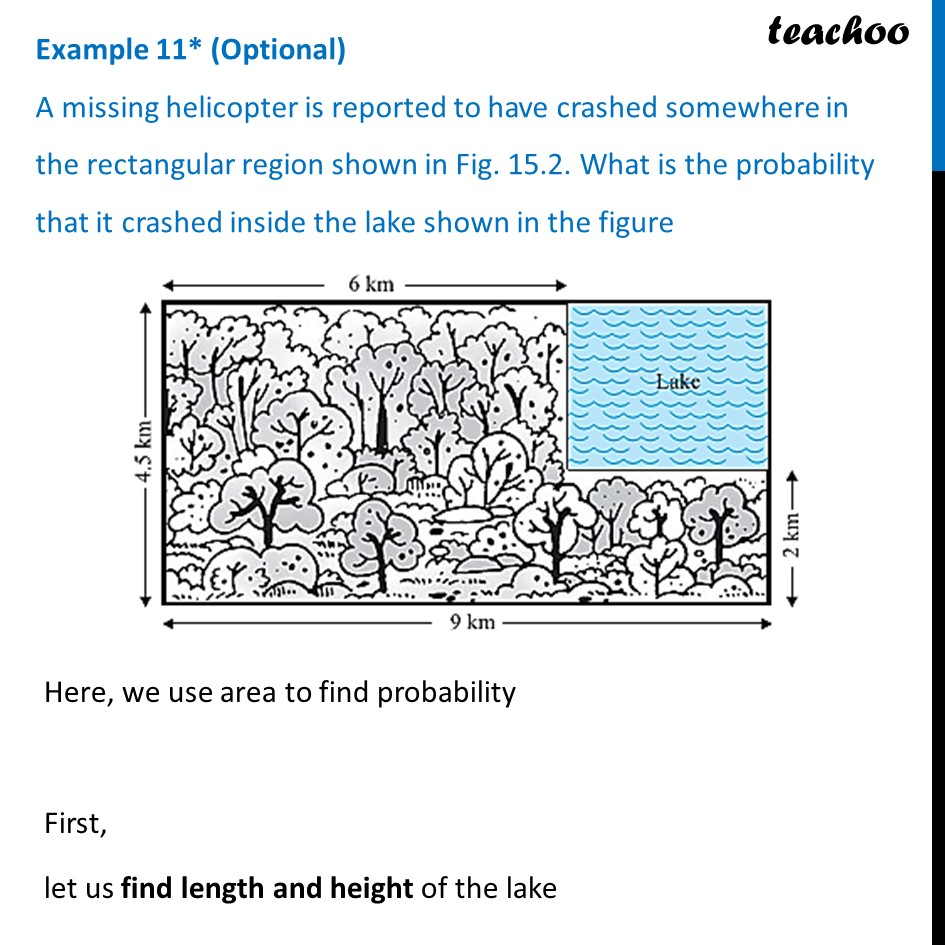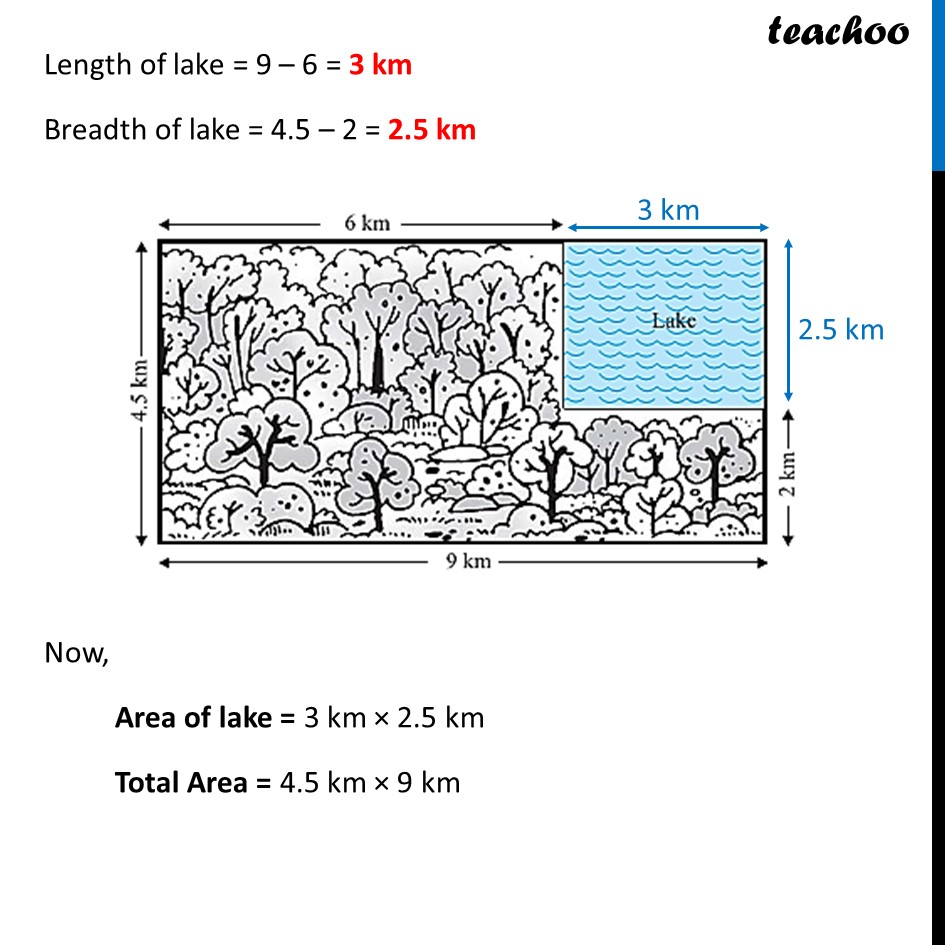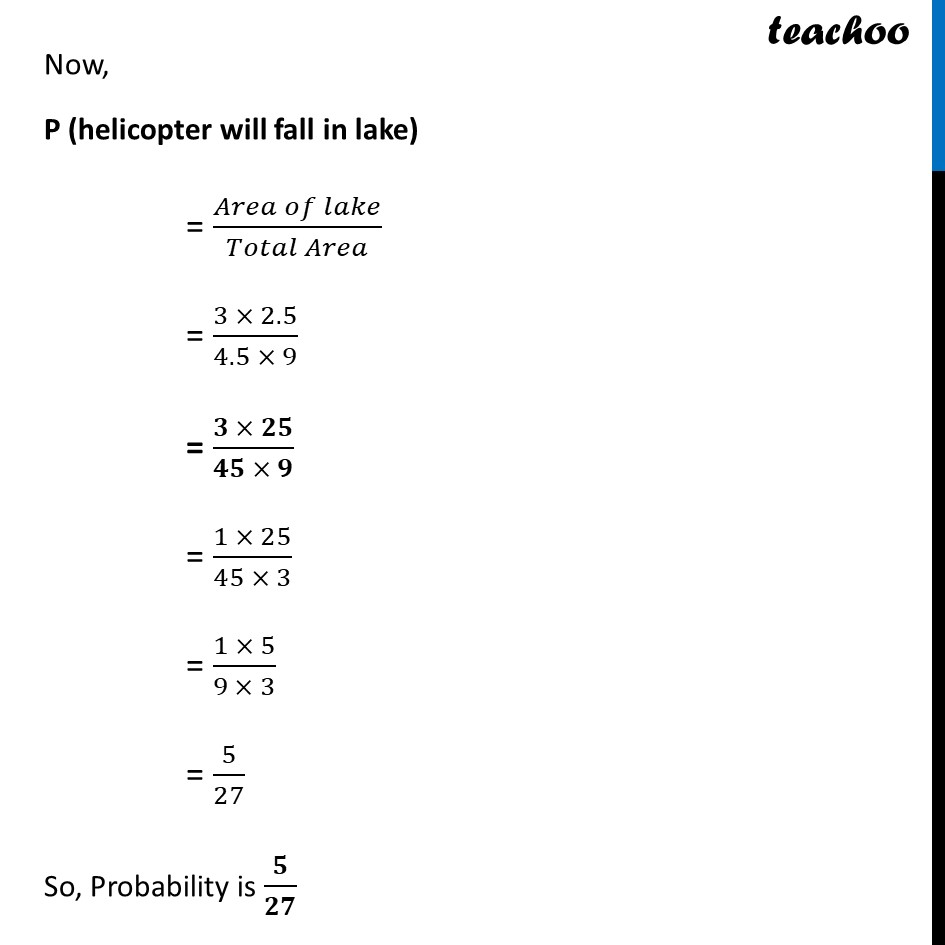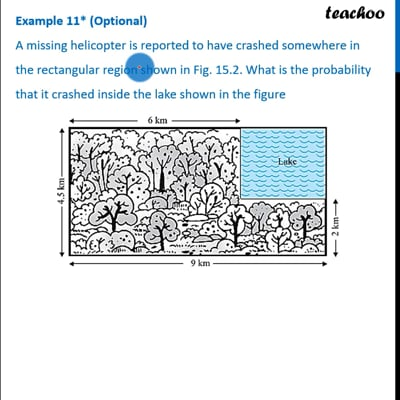Examples

Chapter 15 Class 10 Probability (Term 1)
Serial order wiseThis video is only available for Teachoo black users

### Transcript

Example 11* (Optional) A missing helicopter is reported to have crashed somewhere in the rectangular region shown in Fig. 15.2. What is the probability that it crashed inside the lake shown in the figure Here, we use area to find probability First, let us find length and height of the lake Length of lake = 9 – 6 = 3 km Breadth of lake = 4.5 – 2 = 2.5 km Now, Area of lake = 3 km × 2.5 km Total Area = 4.5 km × 9 km Now, P (helicopter will fall in lake) = (𝐴𝑟𝑒𝑎 𝑜𝑓 𝑙𝑎𝑘𝑒)/(𝑇𝑜𝑡𝑎𝑙 𝐴𝑟𝑒𝑎) = (3 × 2.5)/(4.5 × 9) = (𝟑 × 𝟐𝟓)/(𝟒𝟓 × 𝟗) = (1 × 25)/(45 × 3) = (1 × 5)/(9 × 3) = 5/27 So, Probability is 𝟓/𝟐𝟕